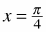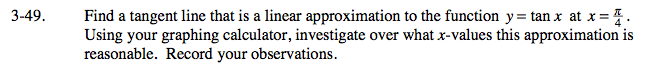### Home > CALC > Chapter 3 > Lesson 3.2.1 > Problem3-49

3-49.

Find a tangent line that is a linear approximation to the function y = tan x at. Using your graphing calculator, investigate over what x-values this approximation is reasonable. Record your observations. Homework Help ✎With your graphing calculator, sketch a graph of y = tanx. Since tan(π/4) = 1, zoom in on the point (π/4, 1) until the graph looks like a straight line. This 'line' is called a 'linear approximation.'

Use your calculator to trace two points on the linear approximation. The more you zoom in, the better. Use those two points to find the equation of the linear approximation.

Input the equation of the linear approximation into your calculator, then zoom out. You will see that the linear approximation models the slope of y = tanx very well near x = π/4. But as you move away from x = π/4, it does not fit as well. On what domain do you think that the linear approximation is a 'good' approximation of the curve?